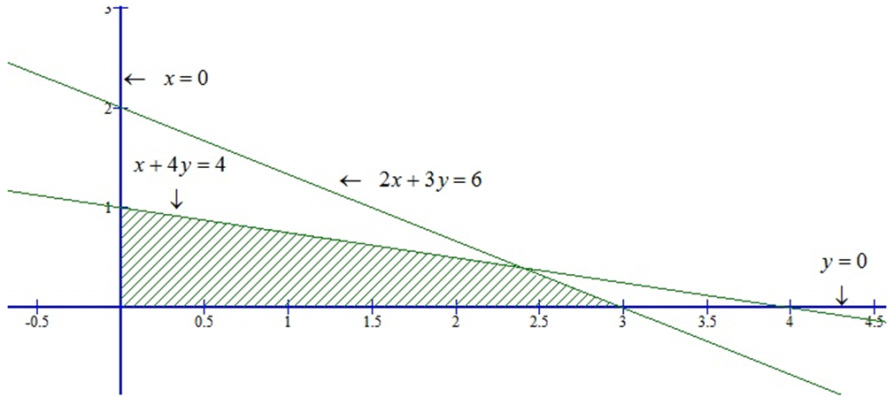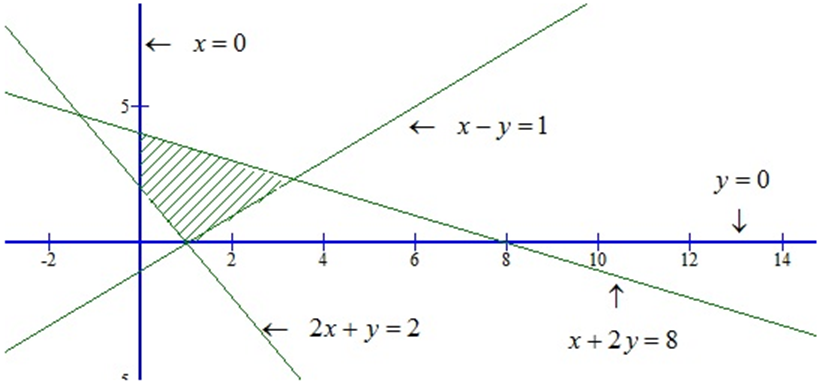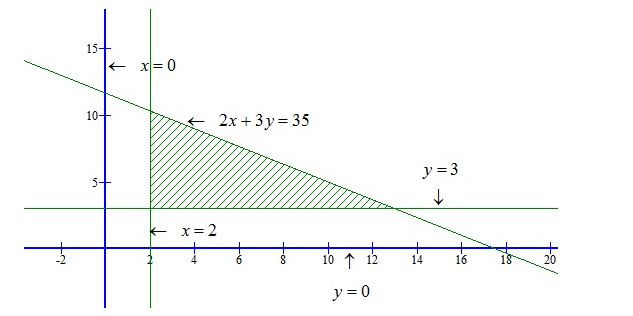×#### Thank you for registering.

One of our academic counsellors will contact you within 1 working day.

Click to Chat

1800-1023-196

+91-120-4616500

CART 0

• 0

MY CART (5)

Use Coupon: CART20 and get 20% off on all online Study Material

ITEM
DETAILS
MRP
DISCOUNT
FINAL PRICE
Total Price: Rs.

There are no items in this cart.
Continue Shopping• Complete JEE Main/Advanced Course and Test Series
• OFFERED PRICE: Rs. 15,900
• View Details

```Chapter 15: Linear Inequations – Exercise 15.6

Linear Inequations – Exercise – 15.6 – Q.1(i)

We have,

2x + 3y ≤ 6,    3x + 2y ≤ 6,    x ≥ 0,  y ≥ 0

Converting the given inequation into equations, the inequations reduce to 2x+ 3y = 6,

3y + 2y = 6, x = 0 and y = 0.

Region represented by 2x + 3y ≤ 6:

Putting x = 0 inequation  2x + 3y = 6

we get y = 6/3 = 2.

Putting y = 0 in the equation 2x + 3y = 6,

we get x = 6/3 = 3.

∴ This line 2x + 3y = 6 meets the coordinate axes at (0, 2) and (3, 0). Draw a thick line joining these points. we find that (0, 0) satisfies inequation 2x + 3y ≤ 6.

Region represented by 3x+ 2y ≤ 6:

Putting x = 0 in the equation

3x + y = 6, we get y = 6/2 = 3.

Putting y = 0 in the equation

3x + y = 6, we get x = 6/2 = 2.

∴ This line 3x + 2y = 6 meets the coordinate axes at (0, 3) and (2, 0). Draw a thick line joining these points. we find that (0,0) satisfies inequation 3x +2y ≤ 6.

Region represented by x ≥ 0 and y ≥ 0:Clearly x ≥ 0 and y ≥ 0 represent the first quadrant.

Linear Inequations – Exercise – 15.6 – Q.1(ii)

We have,

2x+ 3y ≤ 6,    x + 4y ≤ 4, x ≥ 0, y ≥ 0

Converting the inequations into equations, the inequations reduce to 2x+ 3y = 6,

x + 4y = 4, x = 0 and y = 0.

Region represented by 2x+ 3y ≤ 6:

Putting x = 0 in 2x + 3y = 6,

we get y = 6/3 = 2

Putting y = 0 in 2x + 3y = 6,

we get x = 6/2 = 3.

∴ The line 2x + 3y = 6 meets the coordinate axes at (0, 2) and (3, 0). Draw a thick line joining these points. Now, putting x = 0 and y = 0 in 2x+ 3y 6 ⟹ 0 < 6

Clearly, we find that (0, 0) satisfies inequation 2x+ 3y ≤ 6

Region represented by x+ 4y ≤ 4

Putting x = 0 in x + 4y = 4

we get, y = 4/4 = 1

Putting y = 0 in x + 4y = 4,

we get x = 4

∴ The line x + 4y = 4 meets the coordinate axes at (0, 1) and (4, 0). Draw a thick line joining these points. Now, putting x = 0, y = 0

in x + 4y ≤ 4, we get 0 ≤ 4

Clearly, we find that (0, 0) satisfies inequation x + 4y ≤ 4.

Region represented by x ≥ 0 and y ≥ 0:

Clearly x ≥ 0 and y ≥ 0 represent the first quadrant.Linear Inequations – Exercise – 15.6 – Q.1(iii)

We have,

x - y ≤ 1,  x + 2y ≤ 8,   2x + y ≥ 2,  x ≥ 0 and y ≥ 0

Converting the inequations into equations, we obtain

x - y = 1, x + 2y = 8   2x + y = 2, x = 0 and y = 0.

Region represented by x - y ≤ 1:

Putting x = 0 in x - y = 1,

we get y = -1

Putting y = 0 in x - y = 1,

we get x = 1

∴ The line x - y = 1 meets the coordinate axes at (0,-1) and (1, 0). Draw a thick line joining these points. Now putting x = 0 and y = 0 in x - y ≤ 1

in x - y ≤ 1, we get, 0 ≤ 1

Clearly, we find that (0,0) satisfies inequation x - y ≤ 1

Region represented by x + 2 y ≤ 8,

Putting x = 0 in x + 2y = 8,

we get, y = 8/2 = 4

Putting y = 0 in x + 2y = 8,

we get x = 8,

∴ The line x + 2y = 8 meets the coordinate axes at (8, 0) and (0, 4). Draw a thick line joining these points. Now, putting x = 0, y = 0

in x + 2y ≤ 8, we get 0 ≤ 8

Clearly, we find that (0,0) satisfies inequationx + 2y ≤ 8.

Region represented by 2x + y ≥ 2

Putting x = 0 in 2x + y = 2, we get y = 2

Putting y = 0 in 2x + y = 2, we get x = 2/2 = 1.

The line 2x+ y = 2meek the coordinate axes at (0, 2) and (1, 0). Draw a thick line joining these points.Linear Inequations – Exercise – 15.6 – Q.1(iv)

We have,

x + y ≥ 1,   7x + 9y ≤ 63, x ≤ 6,  y ≤ 5 x ≥ 0 and y ≥ 0

Converting the inequations into equations, we obtain

x + y = 1, 7x + 9y = 63   x = 6, y = 5, x = 0 and y = 0.

Region represented by x + y ≥ 1:

Putting x = 0 in x + y = 1, we get y = 1

Putting y = 0 in x + y =1, we get x = 1

∴ The line x + y = 1 meets the coordinate axes at (0,1) and (1,0). join these point by a thick line.

Now putting x = 0 and y = 0 in x + y ≥ 1, we get 0 ≥ 1

This is not possible

∴  (0, 0) is not satisfies the inequality x + y ≥ 1. So, the portion not containing the origin is represented by the inequation x + y ≥ 1.

Region represented by 7X + 9y ≤ 63

Putting x = 0 in 7x + 9y = 63, we get, y = 63/9 = 7.

Putting y = 0 in 7x + 9y = 63, we get x = 63/7 = 9.

∴ The line 7x + 9y = 63 meets the coordinete axes of (0, 7) and (9, 0). Join these points by a thick line. Now putting x = 0 and y = 0

in 7x + 9y ≤ 63, we get, 0 ≤ 63

∴ we find (0, 0) satisfies the inequality 7x + 9y ≤ 63. So, the portion containing the origin represents the solution set of the inequation 7x + 9y ≤ 63.

Region represented by x ≤ 6: Clearly, x = 6 is a line parallel to y-axis at a distance of 6 units from the origin. Since (0, 0) satisfies the inequation x ≤ 6. so, the portion lying on the left side of x = 6 is the region represented by x ≤ 6.

Region represented by y ≤ 5: Clearly, y = 5 is a line parallel to x-axis at a distance 5 from it. since (0, 0) satisfies by the given inequation.

Region represented by x ≥ 0 and y ≥ 0: dearly, x ≥ 0 and y ≥ 0 represent the first quadrant.

The common region of the above six regions represents the solution set of the given inequation as shown below.Linear Inequations – Exercise – 15.6 – Q.1(v)

We have,

2x + 3y ≤ 35,  y ≥ 3,  x ≥ 2, x ≥ 0 and y ≥ 0

Converting the inequations into equations, we get

2x + 3y = 35, y = 3, x = 2, x = 0 and y = 0.

Region represented by 2x + 3y ≤ 35:

Putting x = 0 in 2x + 3y = 35, we get y = 35/3

Putting y = 0 in 2x + 3y = 35, we get x = 35/2

The line 2u +3y = 35 meets the coordinate axes at (0, 35/3)and (35/2, 0). joining these point by

a thick line.

Now, putting x = 0 and y = 0 in 2x + 3y ≤ 35, we get 0 ≤ 35.

Clearly, (0, 0) satisfies the inequality 2x +3y ≤ 35. So, the portion containing the origin represents the solution 2x + 3y ≤ 35.

Region represented by y ≥ 3.

Clearly, y = 3 is a line parallel to x-axis at a distance 3 units from the origin. Since (0,0) does not satisfies the inequation y ≥ 3.

So, the portion not containing the origin is represented by the y ≥ 3.

Region represented by x ≥ 2

Clearly, x = 2 is a line parallel to y-axis at a distance of 2 units from the origin. Since (0,0) does not satisfies the inequation x ≥ 2. so, the portion not containing the origin is represented by the given inequation.

Region represented by x ≥ 0 and y ≥ 0: clearly, x ≥ 0 and y ≥ 0 represent the first quadrant.

The common region of the above five regions represents the solution set of the given inequations as shown below.```### Course Features

• 728 Video Lectures
• Revision Notes
• Previous Year Papers
• Mind Map
• Study Planner
• NCERT Solutions
• Discussion Forum
• Test paper with Video Solution